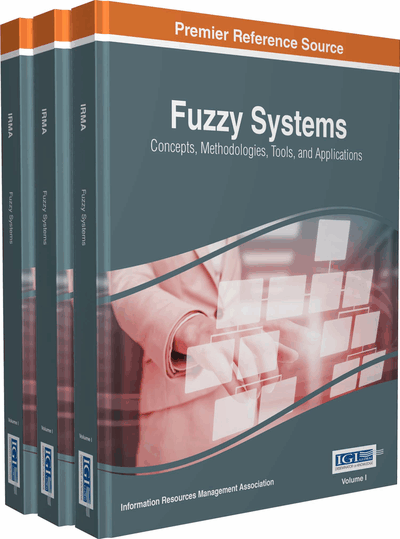# Fuzzy-Based Matrix Converter Drive for Induction Motor

Chitra Venugopal (University of KwaZulu-Natal, South Africa)
DOI: 10.4018/978-1-5225-1908-9.ch033

## Abstract

In industrial applications, approximately, 60% of world's consumption of electrical energy passes through the windings of squirrel-cage induction motors. Hence it is necessary to select an efficient drive circuit for induction motor to save energy. The MC are preferred to replace VSC in industrial applications. To control the performance of the MC, fuzzy logic technique is proposed and simulated using Matlab/Simulink. In this chapter, the basic concepts of MCs are discussed. The implementation of fuzzy logic technique to improve the performance of MC in driving induction motor is discussed in detail. The design of fuzzy controllers and the closed loop control of induction motor is shown. It seen that the introduction of fuzzy controllers in the closed loop helped to reduce the overshoot at starting and maintain the reference speed when running with load torque. Also the input and output voltage of the MC is maintained sinusoidal.
Chapter Preview
Top

## Introduction

Today the world is undergoing a serious power crisis. In industrial applications, variable speed drives plays a vital role in energy saving process. Basically the variable speed drive systems can be classified as DC drive and AC drive systems. The DC drive system uses DC motors which have many disadvantages such as need for regular maintenance, bulky in size and commutator sparking. The AC drive system uses AC motors such as induction or synchronous types. Induction motors are widely used in industrial applications due to their low cost, reliability and performance (Barton, 1994). At present, approximately, 60% of world’s consumption of electrical energy passes through the windings of squirrel-cage induction motors in the range of 1 to 125 horsepower. Hence it is necessary to select a reliable drive circuit for efficient power conversion in variable speed operation of induction motor to save energy.

The speed of the induction motor can be controlled by many techniques such as stator voltage control and frequency control. The frequency control method is best suited for variable speed operation of Induction motors. To perform frequency control method, the power converters should be capable of handling frequency conversion also.

The traditional Voltage Source Converter (VSC) can generate variable voltage and variable frequency ac output from a constant voltage and constant frequency input source. The power conversion in VSC happens in two stages, first stage converts AC into DC and the second stage converts DC into AC with a change in voltage and frequency levels as required. The two stages are linked by an energy storing DC link capacitor. The two stage power conversion compromises the quality of the output power in terms of the harmonics present in the output. Also the bulky DC link capacitor increases the size of the converter. In order to improve the quality of power supplied to the motor, single stage power converters such as cycloconverters and matrix converters are invented. The frequency conversion limitation and the requirement of 72 power switches limit the application of cycloconverters in AC drive system.

Matrix converters are single stage AC to AC power converter. The Matrix converters are called All Silicon Solution because of its ability to perform direct AC to AC frequency and voltage conversion without the need for intermediate energy storage components such as the DC link capacitor as seen in the Voltage Source Converters. Unlike the cycloconverters, matrix converters uses only 9 bidirectional switches arranged in 3x3 matrix form. The Matrix Converters can produce sinusoidal output voltage with controllable displacement factor which increases the quality of power delivered to the stator windings of induction motor. The application of Matrix converter in induction motor drive increases the reliability and performance of the induction motor in speed control applications. The 9 bidirectional switches in 3x3 matrix combination gives 512 (29) switching combinations to produce AC output at required voltage and frequency levels. The switching combinations are selected by modulation methods. The traditional modulation techniques such as space vector and Venturini algorithm are used to control switching combinations of matrix converter.

Though the direct matric converter has several advantages, the application of matrix converter in ac drives especially in the speed control application of induction motor is limited. This is because the implementation of bidirectional switches leads to commutation problems due to the absence of freewheeling paths. This needs to be handled by selecting the proper timing and synchronization of command signals for the switches. The timing and synchronization of switching signal is controlled by modulation and commutation technique. To synchronize the command signals with the switch signals, the current and voltage vectors need to be selected appropriately to select the switching sequence for switches.

Within the last decade, substantial growth of soft computing techniques in electrical drive application has been noticed because the soft computing techniques do not require the mathematical model of the system. The soft computing techniques employ different methods to address the imprecise, uncertain and computation methods of hard computing techniques.

In general, fuzzy logic technique can be used for modelling non-linear, unknown or partially known controllers. It emulates human reasoning providing an intuitive way to design a functional block for a control system. The fuzzy reasoning technique can be utilized to select the current and voltage vectors according to the reference and motor speed of the induction motor.

## Complete Chapter List

Search this Book:
Reset